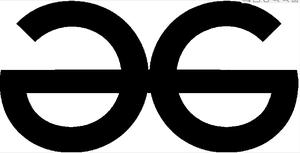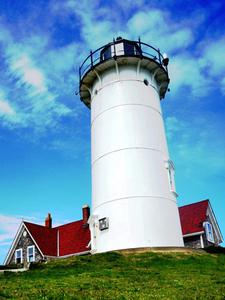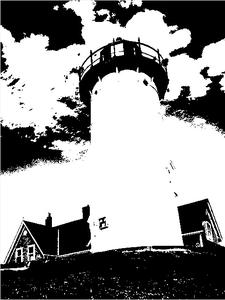GeeksforGeeks App
Open AppBrowser
Continue

# How to Convert RGB Image to Binary Image Using MATLAB?

An Image, by definition, is essentially a visual representation of something  that depicts or records visual perception. Images are classified in one of the three types.

• Binary Images
• Grayscale Images
• Color Images

Binary Images: This is the most basic type of image that exists. The only permissible pixel values in Binary images are 0(Black) and 1(White). Since only two values are required to define the image wholly, we only need one bit and hence binary images are also known as 1-Bit images.

Grayscale Images: Grayscale images are by definition, monochrome images. Monochrome images have only one color throughout and the intensity of each pixel is defined by the gray level it corresponds to. Generally, an 8-Bit image is the followed standard for grayscale images implying that there are 28= 256 grey levels in the image indexed from 0 to 255.

Color Images: Color images can be visualized by 3 color planes(Red, Green, Blue) stacked on top of each other. Each pixel in a specific plane contains the intensity value of the color of that plane. Each pixel in a color image is generally comprised of 24 Bits/pixel with 8 pixels contributing from each color plane.

In this article, we will be discussing how to convert an RGB image to a Binary image using MATLAB.

### Approach :

• Using the im2bw() function in MATLAB, applying thresholding and classifying the pixel values as 0 or 1 by comparing with the threshold.
• Show both the Images together for comparison purposes.

Example 1:

## Matlab

 `% Matlab Code to convert an RGB Image to Binary image``% reading image ``I = imread(``'GFG.jpeg'``);`` ` `% Creating figure window for input image``% Displaying the input image``imshow(I);`` ` `% Converting the image from rgb to binary using thresholding ``J = im2bw(I,0.7);`` ` `% Creating figure window for the output image``% Displaying the output image``imshow(J);`

Output:Figure 1: Input ImageFigure 2 : Output Image

Consider another example with MATLAB’s inbuilt image of a lighthouse.

Example 2:

## Matlab

 `% Matlab Code to convert an RGB Image to Binary image``% reading image ``I = imread(``'lighthouse.png'``);`` ` `% Creating figure window for input image``% Displaying the input image``imshow(I);`` ` `% Converting the image from rgb to ``% binary using thresholding ``J = im2bw(I,0.5);`` ` `% Creating figure window for the output image``% Displaying the output image``imshow(J);`

Output:Figure 3: Input ImageFigure 4: Output Image

Code Explanation: